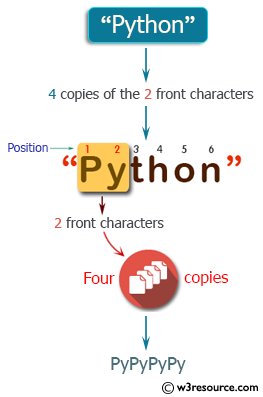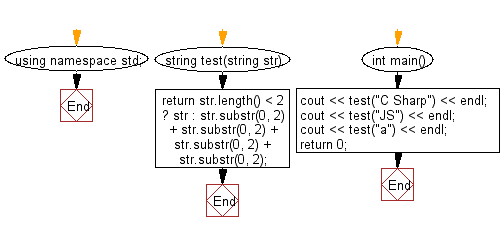﻿ C++ : Four copies of the first two characters of a string# C++ Exercises: Create a new string which is 4 copies of the 2 front characters of a given string

## C++ Basic Algorithm: Exercise-8 with Solution

Write a C++ program to create a new string which is 4 copies of the 2 front characters of a given string. If the given string length is less than 2 return the original string.

Sample Solution:

C++ Code :

``````#include <iostream>
using namespace std;

string test(string str)
{
return str.length() < 2 ? str : str.substr(0, 2) + str.substr(0, 2) + str.substr(0, 2)  + str.substr(0, 2);
}

int main()
{
cout << test("C Sharp") << endl;
cout << test("JS") << endl;
cout << test("a") << endl;
return 0;
}
``````

Sample Output:

```C C C C
JSJSJSJS
a
```

Pictorial Presentation:Flowchart:C++ Code Editor:

Contribute your code and comments through Disqus.

What is the difficulty level of this exercise?

﻿